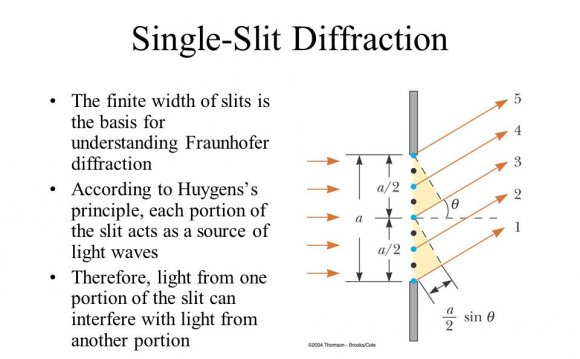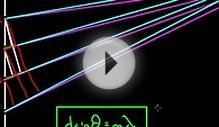# Diffraction of light Waves

May 26, 2022The wave nature of light leads to two very important properties: refraction, where the direction of light propagation is altered at the boundary between media of different densities, and diffraction, which has among its consequences that light can "bend around corners".

### Refraction of Light

The direction of light propagation can be changed at the boundary of two media having different densities. This property is called refraction, and is illustrated in the following figure for the boundary between air and water.

Refraction of light

The apparent and actual positions of the fish differ because the direction of light propagation has been changed as light passes from the more dense water into the less dense air.

If we adopt the convention that the light passes from medium 1 into medium 2, the general rule is that the refraction is

• Away from the perpendicular if medium 2 is less dense than medium 1
• Toward the perpendicular if medium 2 is more dense than medium 1

Thus, in the above example the refraction is away from the perpendicular because air is less dense than water. Such effects form the basis of the refracting telescope, and of optical devices using lenses in general.

### Diffraction of Light

Because light is a wave, it has the capability to "bend around corners". This is called diffraction, and is illustrated in the adjacent image. The intensity of light behind the barrier is not zero in the shadow region. diffractive effects occur generally when a part of a light wave is cut off by an obstruction. Here is a Java applet illustrating diffraction of light by a single slit, and here is an interactive demonstration of refraction and diffraction for ocean waves.

Diffraction has a number of consequences for astronomy. Two of the more important are that this property is the basis for the diffraction grating that can be used to separate light into its constituent colors, and that diffractive effects set an absolute limit on the quality of an image observed through an optical instrument such as a telescope. This diffractive limit occurs because the lenses of such objects are of finite size and diffract light because they cut off part of the light wave.

Back Top Home Help